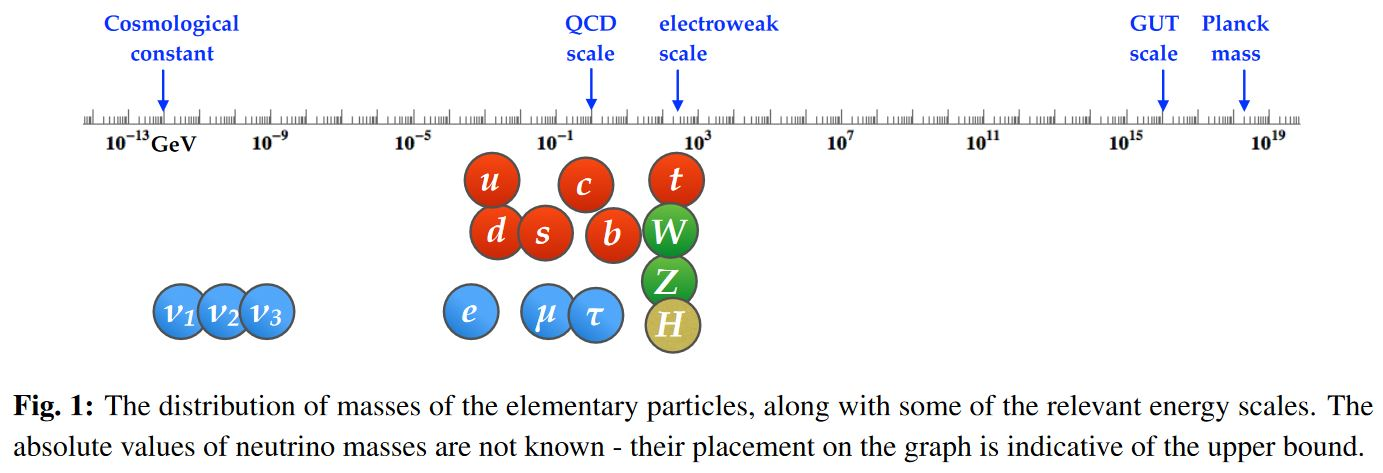Contents

# Contents

## Idea

In physics the scale of the Planck mass (about $10^{19}$ GeV) or Planck length is typically called the Planck scale.

length scales in the observable universe (from cosmic scales, over fundamental particle-masses around the electroweak symmetry breaking to GUT scale and Planck scale):graphics grabbed from Zupan 19

fundamental scales (fundamental physical units)

• speed of light$\,$ $c$

• Planck's constant$\,$ $\hbar$

• gravitational constant$\,$ $G_N = \kappa^2/8\pi$

• Planck scale

• Planck length$\,$ $\ell_p = \sqrt{ \hbar G / c^3 }$

• Planck mass$\,$ $m_p = \sqrt{\hbar c / G}$

• depending on a given mass $m$

• Compton wavelength$\,$ $\lambda_m = \hbar / m c$

• Schwarzschild radius$\,$ $2 m G / c^2$

• depending also on a given charge $e$

• Schwinger limit$\,$ $E_{crit} = m^2 c^3 / e \hbar$
• GUT scale

• string scale

• string tension$\,$ $T = 1/(2\pi \alpha^\prime)$

• string length scale$\,$ $\ell_s = \sqrt{\alpha'}$

• string coupling constant$\,$ $g_s = e^\lambda$